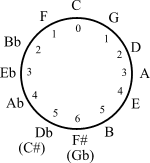# Frequency of Middle C

An educational, fair use website

Bibliographic Entry Result
(w/surrounding text)
Standardized
Result
Henderson, Tom. The Speed of Sound. The Physics Classroom. "Playing middle C on the piano keyboard produces a sound with a frequency of 256 Hz." 256 Hz
Intervals and Notation. The Mathematics of Music. [see editor's supplement below] 278.4375 Hz
Abdullah, T. Yahaya. Musical Scales. Synthesizers, Music & Broadcasting
Tube Length Note Octave Frequency
Original C Original 264 Hz
264 Hz
Culver, C. A. Musical Acoustics. New York: McGraw-Hill, 1956. "Some scientific manufacturers once adopted a standard of 256 Hz for middle C, but musicians ignored it." 256 Hz
Maths & Music - Frequency. Philharmonic Physics. "The note musicians call Middle C has a frequency of 262Hz." 262 Hz

In music there is an octave that is made up of eight notes. It goes C, D, E, F, G, A, B, C, and continues up and down on a standard musical instrument. The middle A, above middle C, has a frequency of 440 Hz. To find the frequency of a note an octave higher the frequency is doubled. To find the frequency of a note one octave lower the frequency is halved.

Frequency can also go in fifths by using the formula x(3/2) = y where x is the original note and y is the fifth note above the original. Follow the octave going up and down where needed. The frequency of middle C turns out to be 278.4375 Hz. [See editor's supplement below.]

There are different types of scales. For example just intonation, equal temperament, mean tempered, American standard, and international standard. Scales are built on ratios and fixed notes. A different set of ratios and a different fixed pitch will result in a different value for middle C.

Since each scale has a different frequency for middle C, the frequency has also been known as 262 Hz, 256 Hz, and 264 Hz. The frequency for one trial comes out to be 262 Hz because the frequency of the C one octave higher than middle C in that scale is 524 Hz. In order to find the frequency of a note one octave lower the frequency is halved. In general, the frequency of middle C is between 256 Hz and 280 Hz.

Danielle Daly -- 2003

Bibliographic Entry Result
(w/surrounding text)
Standardized
Result
Circle of Fifths, Wikipedia, 2005264.298 Hz
[see explanation below]

The circle of fifths is a method for generating a musical scale that is often credited to the Classical Greek mathematician Pythagoras. Start with a note you like and multiply it by 3/2. This ratio is called a fifth. If the resulting note is too high, divide it by two. Multiplying and dividing by two changes the octave of the note. Keep repeating this over and over again until you have all twelve notes of the European chromatic scale — the 7 notes corresponding to the letters A through G plus 5 extra notes midway between the original 7 indicated with the addition of a sharp (♯) or flat (♭) symbol. The order in which this method generates notes is written clockwise around the circumference of a circle; thus the name "circle of fifths".

The student who wrote the essay above cites a page called Intervals and Notation that claims that C can be generated from A by the following procedure…

 A-almost-an-octave-above-middle-C is at 440 Hz Raise a fifth to E-more-than-an-octave-above-middle-C 440(3/2)=660 Hz Lower an octave to E-just-above-middle-C 660/2=330Hz Raise a fifth to B-almost-an-octave-above-middle-C 330(3/2)=495 Lower one octave to B-immediately-below-middle-C 495/2=247.5Hz Raise a fifth to F-immediately-above-middle-C 247.5(3/2)=371.25 Hz Lower an octave to F-below-middle-C 371.25/2=185.625 Hz Raise a fifth to Middle C 185.625(3/2)=278.4375 Hz Therefore, Middle C is at a frequency of 278.4375 Hz

This is not correct. Acccording to the circle of fiths, to get to C from A requires a jump of 9 fifths up.

440 Hz * (3/2)^9 = 16,915 Hz

Stepping down 6 octaves gives us a value in the desired range.

16,915Hz/2^6 = 264.298 Hz

Since the author (or authors) of Intervals and Notation jumped up 4 fifths, what they probably did was start at the A flat (A♭) position on the circle by mistake. Or perhaps they did not realize that the last jump at B would take them to F♯, not F.

Editor's Supplement -- 2006